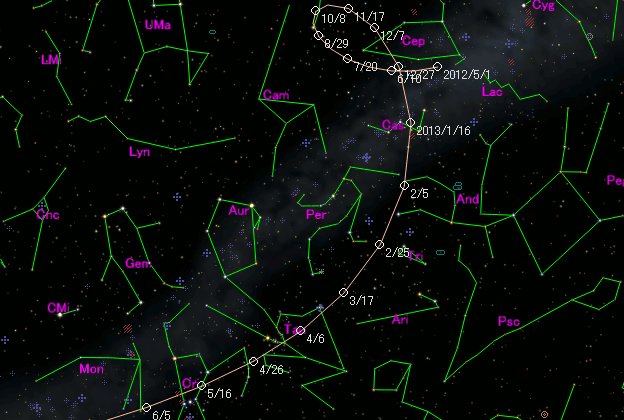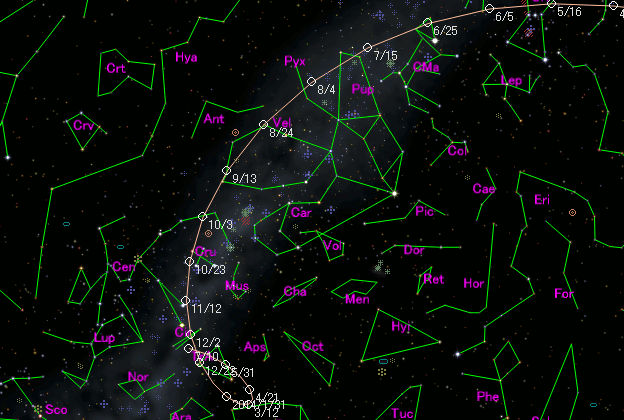# \$B%j%K%"WB@1(B

C/2012 L2 ( LINEAR )###\$B%W%m%U%#!<%k(B

 \$BH/8+F|(B 2012\$BG/(B6\$B7n(B1\$BF|(B \$BH/8+8wEY(B 19.4\$BEy(B \$BH/8+ Lincoln Laboratory Near-Earth Asteroid Research project

###\$B###\$B50F;MWAG(B

```Epoch 2013 May 28.0 TT = JDT 2456440.5
T 2013 May  9.32260 TT                                  MPC
q   1.5086427            (2000.0)            P               Q
z  +0.0017618      Peri.  205.77632     -0.14643666     -0.29114459
+/-0.0000006      Node   270.30133     +0.98903171     -0.06173899
e   0.9973421      Incl.   70.98237     -0.01930226     -0.95468483
From 2048 observations 2012 June 1-2013 Oct. 12, mean residual 0".5.
```

###\$B@1?^(B###\$B8wEYJQ2=(B

```        m1 = 7.0 + 5 log\$B&\$(B + 12.5 log r  [   ,-58]  (              \$B!A(B2013\$BG/(B 3\$B7n(B12\$BF|(B)
m1 = 3.5 + 5 log\$B&\$(B + 27.5 log r  [-58,   ]  (2013\$BG/(B 3\$B7n(B12\$BF|!A(B              )
```##### \$B50F;MWAG\$O(BM.P.E.C. 2014-H26\$B\$K7G:\\$5\$l\$?\$b\$N\$G\$9!#(B \$B@1?^\$O%9%F%i%J%S%2!<%?(B Ver.8 (\$B%"%9%H%m%"!<%D(B) \$B\$G:n@.\$7\$?\$b\$N\$G\$9!#(B \$B8wEY%0%i%U\$O(BComet for Windows\$B\$G:n@.\$7\$?\$b\$N\$G\$9!#(B